# 红黑树（附完整C代码）

## 红黑树简介### 用途

• STL（标准模板库）中在set map是基于红黑树实现的。
• Java中在TreeMap使用的也是红黑树。
• epoll在内核中的实现，用红黑树管理事件块。
• linux进程调度Completely Fair Scheduler,用红黑树管理进程控制块

### 红黑树 VS AVL树

1. 从实现细节上来讲，如果插入一个结点引起了树的不平衡，AVL树和红黑树都最多需要2次旋转操作，即两者都是O(1)；但是在删除node引起树的不平衡时，最坏情况下，AVL需要维护从被删node到root这条路径上所有node的平衡性，因此需要旋转的量级O(logN)，而RB-Tree最多只需3次旋转，只需要O(1)的复杂度

2. 从两种平衡树对平衡的要求来讲，AVL的结构相较RB-Tree来说更为平衡，在插入和删除node更容易引起Tree的unbalance，因此在大量数据需要插入或者删除时，AVL需要rebalance的频率会更高。因此，RB-Tree在需要大量插入和删除node的场景下，效率更高。自然，由于AVL高度平衡，因此AVL的search效率更高。

3. 总体来说，RB-tree的统计性能是高于AVL的。

## 关于参考书

《算法导论》和《数据结构与算法分析》是大家常用的两本算法书，针对红黑树这一章，这两本书上也都有，但是二者从数据结构到使用的方法上都不一样，这里我推荐使用《算法导论》。有以下几个原因：

• 《数据结构与算法分析》中使用的数据结构没有保存父亲结点，所以在调用旋转函数时需要用函数返回值来保持上下结点的连接，这在avl树中显得很简洁，因为在avl中的情况比较简单，但是在红黑树中涉及到了祖祖父、祖父、父亲、儿子、四代结点，按照这本书上的方法得保存GGP、GP、P、X四个全局变量的值，在更新它们的指向时非常容易搞混。
• 《数据结构与算法分析》没有实现删除操作，只是描述了实现的方法，按照这上面的方法我也做了实现，最后发现代码非常长，至少150+，不如《算法导论》中的简洁。
• 另外，《算法导论》中描述的方法比较完整，思路严谨。

## 红黑树详解

### 红黑树性质

1. 列表项结点是红色或黑色。
2. 根是黑色。
3. 所有叶子都是黑色（叶子是NIL结点）。
4. 每个红色结点必须有两个黑色的子结点。（从每个叶子到根的所有路径上不能有两个连续的红色结点。）
5. 从任一结点到其每个叶子的所有简单路径都包含相同数目的黑色结点。
为了便于处理红黑树中的边界情况，使用一个哨兵来代表所有的NIL结点，也就是说所有指向NIL的指针都指向哨兵T.nil。

### 红黑树数据结构

typedef enum ColorType {RED, BLACK} ColorType;
typedef struct rbt_t{
int key;
rbt_t * left;
rbt_t * right;
rbt_t * p;
ColorType color;
}rbt_t;

typedef struct rbt_root_t{
rbt_t* root;
rbt_t* nil;
}rbt_root_t;

/*
*@brief rbt_init 初始化
*/
rbt_root_t* rbt_init(void){
rbt_root_t* T;

T = (rbt_root_t*)malloc(sizeof(rbt_root_t));
assert( NULL != T);

//用一个哨兵代表NIL。
T->nil = (rbt_t*)malloc(sizeof(rbt_t));
assert(NULL != T->nil);
T->nil->color = BLACK;
T->nil->left = T->nil->right = NULL;
T->nil->p = NULL;

T->root = T->nil;
return T;
}

### 红黑树旋转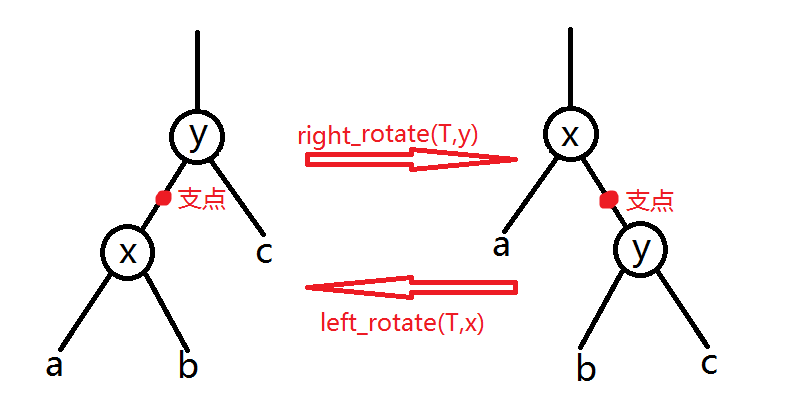c代码：

/*
*@brief rbt_left_rotate
*@param[in] T 树根
*@param[in] x 要进行旋转的节点
*/
void rbt_left_rotate( rbt_root_t* T, rbt_t* x){
rbt_t* y = x->right;

x->right = y->left;
if(x->right != T->nil)//更新某结点的父亲时，要确定此结点不是T.nil
x->right->p = x;

y->p = x->p;
if(x->p == T->nil){//如果x以前是树根，那么现在树根易主了
T->root = y;
}else if(y->key < y->p->key)
y->p->left = y;
else
y->p->right = y;

y->left = x;
x->p = y;
}
/*
*@brief rbt_right_rotate
*@param[in] 树根
*@param[in] 要进行旋转的节点
*/
void rbt_right_rotate( rbt_root_t* T, rbt_t* x){
rbt_t * y = x->left;
x->left = y->right;

if(T->nil != x->left)
x->left->p = x;

y->p = x->p;
if(y->p == T->nil)
T->root = y;
else if(y->key < y->p->key)
y->p->left= y;
else
y->p->right = y;

y->right = x;
x->p = y;
}

### 红黑树插入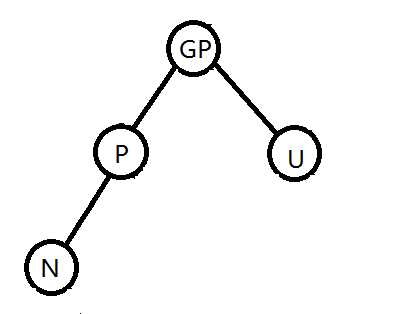1. 新插入的节点一定是红色的。（如果是黑色的，会破坏条件5）
2. 如果新插入的节点的父亲是黑色的，则没有破坏任何性质，那么插入完成。
3. 如果插入节点的父节点是红色, 破坏了性质4. 故插入算法就是通过重新着色或旋转, 来维持性质

N为红，P为红（GP一定为黑），U为红。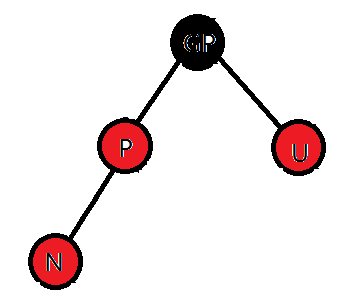N，P都为红（GP一定为黑)，U为黑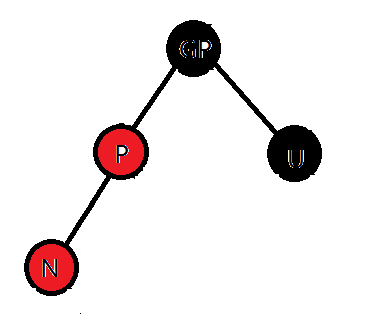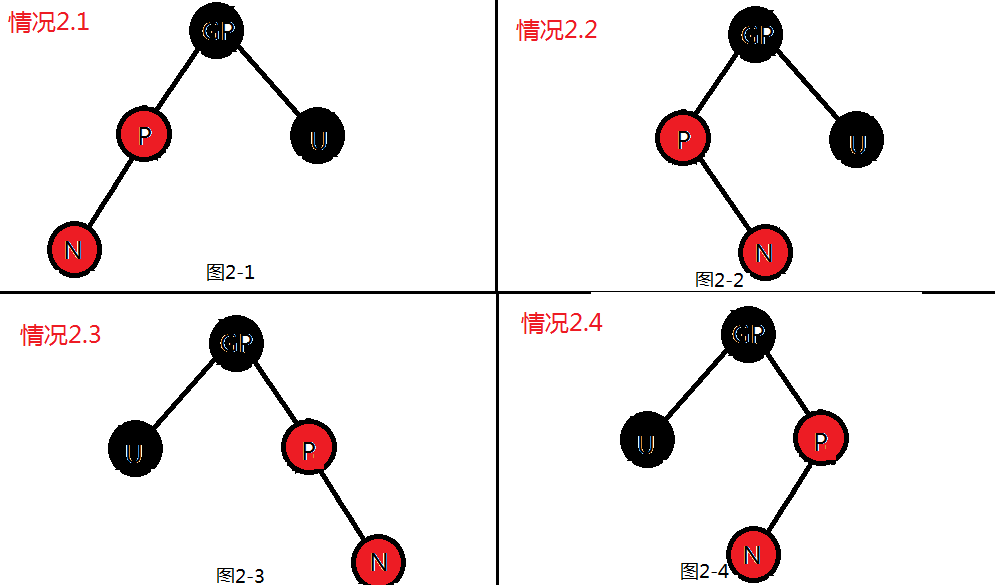### 红黑树删除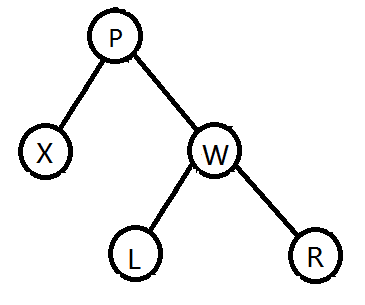void rbt_transplant(rbt_root_t* T, rbt_t* u, rbt_t* v){
if(u->p == T->nil)
T->root = v;
else if(u == u->p->left)
u->p->left =v;
else
u->p->right = v;
v->p = u->p;//即使v是T.nil也可以执行这一行
}

x的兄弟w是红色的，那么它们的父亲、w的孩子都是黑色的。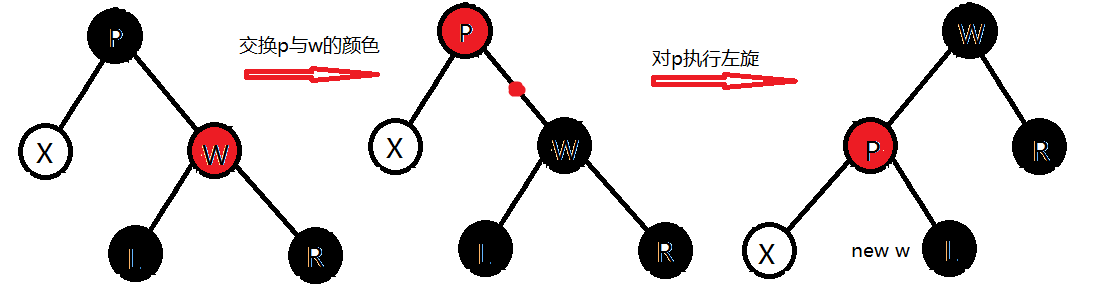x的兄弟w是黑色，而且w的两个孩子都是黑色。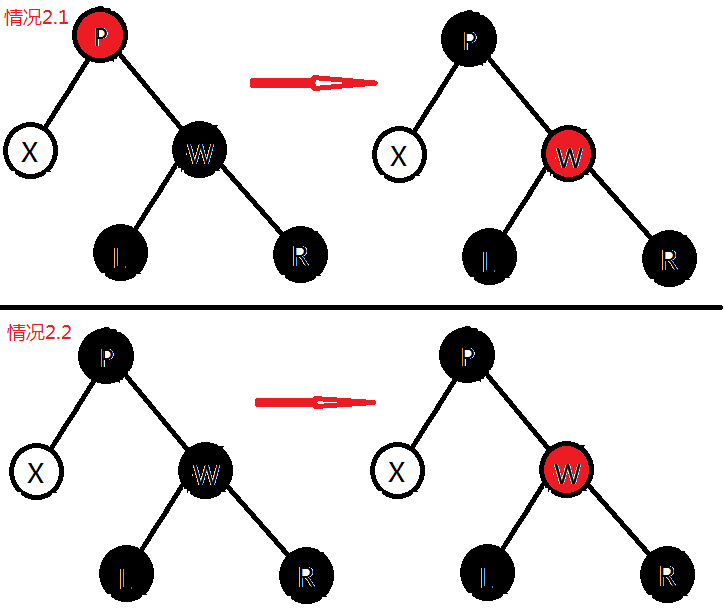W是黑色有，w在左孩子是红色的，W的右孩子是黑色的。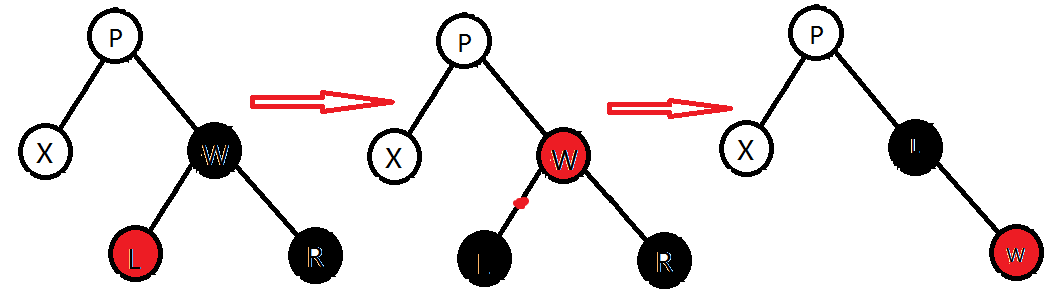w是黑色的，w的右孩子是红色的。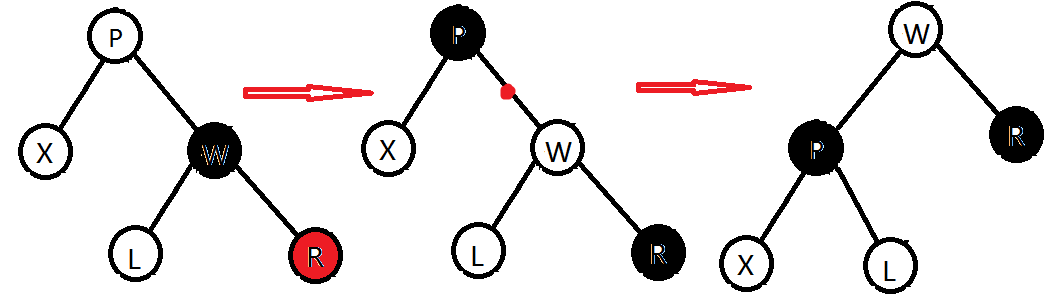## 完整代码（C）

#include<stdafx.h>
#include<malloc.h>
#include <assert.h>

//版权声明：原创不易，转载请注明转自[weewqrer 红黑树](http://blog.csdn.net/weewqrer/article/details/51866488)

//红黑树
typedef enum ColorType {RED, BLACK} ColorType;
typedef struct rbt_t{
int key;
rbt_t * left;
rbt_t * right;
rbt_t * p;
ColorType color;
}rbt_t;

typedef struct rbt_root_t{
rbt_t* root;
rbt_t* nil;
}rbt_root_t;

//函数声明
rbt_root_t* rbt_init(void);
static void rbt_handleReorient(rbt_root_t* T, rbt_t* x, int k);
rbt_root_t* rbt_insert(rbt_root_t* &T, int k);
rbt_root_t* rbt_delete(rbt_root_t* &T, int k);

void rbt_transplant(rbt_root_t* T, rbt_t* u, rbt_t* v);

static void rbt_left_rotate( rbt_root_t* T, rbt_t* x);
static void rbt_right_rotate( rbt_root_t* T, rbt_t* x);

void rbt_inPrint(const rbt_root_t* T, rbt_t* t);
void rbt_prePrint(const rbt_t * T, rbt_t* t);
void rbt_print(const rbt_root_t* T);

static rbt_t* rbt_findMin(rbt_root_t * T, rbt_t* t);
static rbt_t* rbt_findMax(rbt_root_t * T, rbt_t* t);

static rbt_t* rbt_findMin(rbt_root_t * T, rbt_t* t){
if(t == T->nil) return T->nil;

while(t->left != T->nil)
t = t->left;
return t;
}
static rbt_t* rbt_findMax(rbt_root_t * T, rbt_t* t){
if(t == T->nil) return T->nil;

while(t->right != T->nil)
t = t->right;
return t;
}
/*
*@brief rbt_init 初始化
*/
rbt_root_t* rbt_init(void){
rbt_root_t* T;

T = (rbt_root_t*)malloc(sizeof(rbt_root_t));
assert( NULL != T);

T->nil = (rbt_t*)malloc(sizeof(rbt_t));
assert(NULL != T->nil);
T->nil->color = BLACK;
T->nil->left = T->nil->right = NULL;
T->nil->p = NULL;

T->root = T->nil;

return T;
}

/*
*@brief rbt_handleReorient  内部函数 由rbt_insert调用
*      在两种情况下调用这个函数：
* 1 x有连个红色儿子
* 2 x为新插入的结点
*
*/
void rbt_handleReorient(rbt_root_t* T, rbt_t* x, int k){

//在第一种情况下，进行颜色翻转； 在第二种情况下，相当于对新插入的x点初始化
x->color = RED;
x->left->color = x->right->color = BLACK;

//如果x.p为红色，那么x.p一定不是根，x.p.p一定不是T.nil，而且为黑色
if(  RED == x->p->color){
x->p->p->color = RED;//此时x, p, x.p.p都为红

if(x->p->key < x->p->p->key){
if(k > x->p->key){
x->color = BLACK;//小心地处理颜色
rbt_left_rotate(T,x->p);
rbt_right_rotate(T,x->p);
}else{
x->p->color = BLACK;//小心地处理颜色
rbt_right_rotate(T,x->p->p);
}

}else{
if(k < x->p->key){
x->color = BLACK;
rbt_right_rotate(T,x->p);
rbt_left_rotate(T,x->p);
}else{
x->p->color = BLACK;
rbt_left_rotate(T,x->p->p);
}

}
}

T->root->color = BLACK;//无条件令根为黑色
}
/*
*@brief brt_insert 插入
*1 新插入的结点一定是红色的，如果是黑色的，会破坏条件4（每个结点到null叶结点的每条路径有同样数目的黑色结点）
*2 如果新插入的结点的父亲是黑色的，那么插入完成。 如果父亲是红色的，那么做一个旋转即可。（前提是叔叔是黑色的）
*3 我们这个插入要保证其叔叔是黑色的。也就是在x下沉过程中，不允许存在两个红色结点肩并肩。
*/
rbt_root_t* rbt_insert(rbt_root_t* &T, int k){

rbt_t * x, *p;
x = T->root;
p = x;

//令x下沉到叶子上，而且保证一路上不会有同时为红色的兄弟
while( x != T->nil){
//
//保证没有一对兄弟同时为红色， 为什么要这么做？
if(x != T->nil)
if(x->left->color == RED && x->right->color == RED)
rbt_handleReorient(T,x,k);

p = x;
if(k<x->key)
x = x->left;
else if(k>x->key)
x = x->right;
else{
printf("\n%d已存在\n",k);
return T;
}

}

//为x分配空间，并对其进行初始化
x = (rbt_t *)malloc(sizeof(rbt_t));
assert(NULL != x);
x->key = k;
x->color = RED;
x->left = x->right = T->nil;
x->p = p;

//让x的父亲指向x
if(T->root == T->nil)
T->root = x;
else if(k < p->key)
p->left = x;
else
p->right = x;

//因为一路下来，如果x的父亲是红色，那么x的叔叔肯定不是红色了，这个时候只需要做一下翻转即可。
rbt_handleReorient(T,x,k);

return T;
}
void rbt_transplant(rbt_root_t* T, rbt_t* u, rbt_t* v){
if(u->p == T->nil)
T->root = v;
else if(u == u->p->left)
u->p->left =v;
else
u->p->right = v;
v->p = u->p;
}
/*
*@brief rbt_delete 从树中删除 k
*
*
*/
rbt_root_t* rbt_delete(rbt_root_t* &T, int k){
assert(T != NULL);
if(NULL == T->root) return T;

//找到要被删除的叶子结点
rbt_t * toDelete = T->root;
rbt_t * x;

//找到值为k的结点
while(toDelete != T->nil && toDelete->key != k){
if(k<toDelete->key)
toDelete = toDelete->left;
else if(k>toDelete->key)
toDelete = toDelete->right;
}

if(toDelete == T->nil){
printf("\n%d 不存在\n",k);
return T;
}

//如果两个孩子，就找到右子树中最小的代替, alternative最多有一个右孩子
if(toDelete->left != T->nil && toDelete->right != T->nil){
rbt_t* alternative = rbt_findMin(T, toDelete->right);
k = toDelete->key = alternative->key;
toDelete = alternative;
}

if(toDelete->left == T->nil){
x = toDelete->right;
rbt_transplant(T,toDelete,toDelete->right);
}else if(toDelete->right == T->nil){
x = toDelete->left;
rbt_transplant(T,toDelete,toDelete->left);
}

if(toDelete->color == BLACK){
//x不是todelete，而是用于代替x的那个
//如果x颜色为红色的，把x涂成黑色即可， 否则 从根到x处少了一个黑色结点，导致不平衡
while(x != T->root && x->color == BLACK){
if(x == x->p->left){
rbt_t* w = x->p->right;

//情况1 x的兄弟是红色的，通过
if(RED == w->color){
w->color = BLACK;
w->p->color = RED;
rbt_left_rotate(T,x->p);
w = x->p->right;
}//处理完情况1之后，w.color== BLACK ， 情况就变成2 3 4 了

//情况2 x的兄弟是黑色的，并且其儿子都是黑色的。
if(w->left->color == BLACK && w->right->color == BLACK){
if(x->p->color == RED){
x->p->color = BLACK;
w->color = RED;

break;
}else{
w->color = RED;
x = x->p;//x.p左右是平衡的，但是x.p处少了一个黑结点，所以把x.p作为新的x继续循环
continue;
}
}

//情况3 w为黑色的，左孩子为红色。（走到这一步，说明w左右不同时为黑色。）
if(w->right->color == BLACK){
w->left->color = BLACK;
w->color = RED;
rbt_right_rotate(T,w);
w = x->p->right;
}//处理完之后，变成情况4

//情况4 走到这一步说明w为黑色， w的左孩子为黑色， 右孩子为红色。

w->color=x->p->color;
x->p->color=BLACK;
w->right->color=BLACK;
rbt_left_rotate(T,x->p);
x = T->root;
}else{
rbt_t* w = x->p->left;
//1
if(w->color == RED){
w->color = BLACK;
x->p->color = RED;
rbt_right_rotate(T,x->p);
w = x->p->left;
}
//2
if(w->left->color==BLACK && w->right->color == BLACK){
if(x->p->color == RED){
x->p->color = BLACK;
w->color = RED;
break;
}else{
x->p->color = BLACK;
w->color = RED;
x = x->p;
continue;
}
}

//3
if(w->left->color == BLACK){
w->color = RED;
w->right->color = BLACK;
w = x->p->left;
}

//4
w->color=w->p->color;
x->p->color = BLACK;
w->left->color = BLACK;
rbt_right_rotate(T,x->p);
x = T->root;
}

}
x->color = BLACK;
}

//放心删除todelete 吧
free(toDelete);

return T;
}

/*
*@brief rbt_left_rotate
*@param[in] T 树根
*@param[in] x 要进行旋转的结点
*/
void rbt_left_rotate( rbt_root_t* T, rbt_t* x){
rbt_t* y = x->right;

x->right = y->left;
if(x->right != T->nil)
x->right->p = x;

y->p = x->p;
if(y->p == T->nil){
T->root = y;
}else if(y->key < y->p->key)
y->p->left = y;
else
y->p->right = y;

y->left = x;
x->p = y;
}
/*
*@brief rbt_right_rotate
*@param[in] 树根
*@param[in] 要进行旋转的结点
*/
void rbt_right_rotate( rbt_root_t* T, rbt_t* x){
rbt_t * y = x->left;
x->left = y->right;

if(T->nil != x->left)
x->left->p = x;

y->p = x->p;
if(y->p == T->nil)
T->root = y;
else if(y->key < y->p->key)
y->p->left= y;
else
y->p->right = y;

y->right = x;
x->p = y;
}
void rbt_prePrint(const rbt_root_t* T, rbt_t* t){
if(T->nil == t)return ;
if(t->color == RED)
printf("%3dR",t->key);
else
printf("%3dB",t->key);
rbt_prePrint(T,t->left);
rbt_prePrint(T,t->right);
}
void rbt_inPrint(const rbt_root_t* T, rbt_t* t){
if(T->nil == t)return ;
rbt_inPrint(T,t->left);
if(t->color == RED)
printf("%3dR",t->key);
else
printf("%3dB",t->key);
rbt_inPrint(T,t->right);
}

//打印程序包括前序遍历和中序遍历两个，因为它俩可以唯一确定一棵二叉树
void rbt_print(const rbt_root_t* T){
assert(T!=NULL);
printf("\n前序遍历 ：");
rbt_prePrint(T,T->root);
printf("\n中序遍历 ：");
rbt_inPrint(T,T->root);
printf("\n");
}

void rbt_test(){
rbt_root_t* T = rbt_init();

/************************************************************************/
/* 1    测试插入
/*
/*
/*输出  前序遍历 ：  7B  2R  1B  5B  4R 11R  8B 14B 15R
/*      中序遍历 ：  1B  2R  4R  5B  7B  8B 11R 14B 15R
/************************************************************************/

T = rbt_insert(T,11);
T = rbt_insert(T,7);
T = rbt_insert(T,1);
T = rbt_insert(T,2);
T = rbt_insert(T,8);
T = rbt_insert(T,14);
T = rbt_insert(T,15);
T = rbt_insert(T,5);
T = rbt_insert(T,4);

T = rbt_insert(T,4); //重复插入测试
rbt_print(T);

/************************************************************************/
/* 2    测试删除
/*
/*操作  连续删除4个元素 rbt_delete(T,8);rbt_delete(T,14);rbt_delete(T,7);rbt_delete(T,11);
/*输出  前序遍历 ：  2B  1B  5R  4B 15B
/*      中序遍历 ：  1B  2B  4B  5R 15B
/************************************************************************/

rbt_delete(T,8);
rbt_delete(T,14);rbt_delete(T,7);rbt_delete(T,11);

rbt_delete(T,8);//删除不存在的元素
rbt_print(T);

}



12-20245
10-082625
01-16832
06-292080
09-02267
08-103433
11-247629
05-231442
08-148443
05-19448
04-252328
10-19437
09-01213
09-2170
08-18352
01-03238
01-197708
09-01406
05-081万+
09-27102

### “相关推荐”对你有帮助么？

•非常没帮助
•没帮助
•一般
•有帮助
•非常有帮助被折叠的  条评论 为什么被折叠?到【灌水乐园】发言weewqrer

¥2 ¥4 ¥6 ¥10 ¥20余额支付 (余额：-- )扫码支付获取中扫码支付点击重新获取扫码支付1.余额是钱包充值的虚拟货币，按照1:1的比例进行支付金额的抵扣。
2.余额无法直接购买下载，可以购买VIP、C币套餐、付费专栏及课程。余额充值